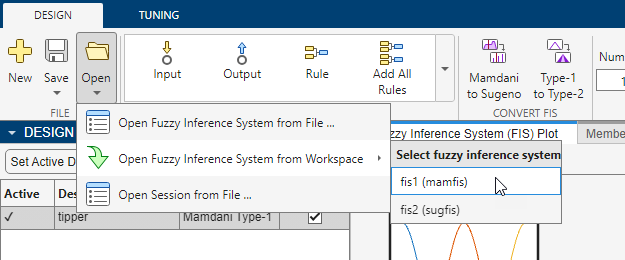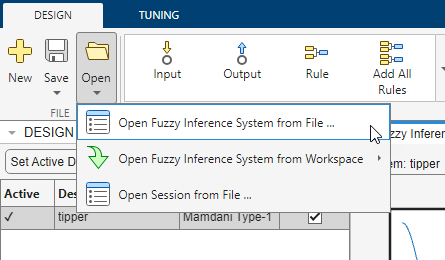# Fuzzy Logic Designer

Design, test, and tune fuzzy inference systems

## Description

The Fuzzy Logic Designer app lets you design, test, and tune a fuzzy inference system (FIS) for modeling complex system behavior.

Using this app, you can:

• Design Mamdani and Sugeno FISs.

• Design type-1 and type-2 FISs.

• Tune the rules and membership functions of a FIS.

• Add or remove input and output variables.

• Specify input and output membership functions.

• Define fuzzy if-then rules.

• Select fuzzy inference functions for:

• And operations

• Or operations

• Implication

• Aggregation

• Defuzzification

• Analyze the behavior of a FIS, including:

• View rule inference process for given input values.

• View output surface maps for fuzzy inference systems.

• Compare FIS outputs with corresponding output values from testing data.

• View error distributions across input ranges based on testing data.

• Export FIS designs to the MATLAB® workspace.

### Limitations

The Fuzzy Logic Designer app does not support the following tasks.

• FIS tree creation — Instead, create FIS trees at the MATLAB command line using `fistree` objects. You can create the component FIS objects using Fuzzy Logic Designer and export them to the MATLAB workspace.

• FIS tree tuning — Instead, tune FIS trees at the MATLAB command line using the `tunefis` function. You can tune the component FIS objects using Fuzzy Logic Designer and export them to the MATLAB workspace.

## Open the Fuzzy Logic Designer App

• MATLAB Toolstrip: On the Apps tab, under Control System Design and Analysis, click the app icon.

• MATLAB command prompt: Enter `fuzzyLogicDesigner`.

## Examples

expand all

In Fuzzy Logic Designer, under Import > Import Fuzzy Inference System from Workspace, click the fuzzy inference system.A new Fuzzy Logic Designer instance opens and loads the selected FIS.

In Fuzzy Logic Designer, select Import > Import Fuzzy Inference System from Workspace.Then, in the Import Fuzzy Inference System dialog box, select a FIS file and click .

Using Fuzzy Logic Designer, you can convert between Mamdani and Sugeno systems and between type-1 and type-2 systems.

When you convert a FIS, the app adds the converted FIS to the Design Browser. To make the converted system active, select it in the Design Browser and click .

For more information on converting between type-1 and type2 systems, see `convertToType1` and `convertToType2`.

For more information on converting between Mamdani and Sugeno systems, see Mamdani and Sugeno Fuzzy Inference Systems.

## Programmatic Use

expand all

`fuzzyLogicDesigner` opens the Fuzzy Logic Designer app and loads the Getting Started dialog box where you can open an existing FIS or create an initial FIS structure. For more information, see Get Started Using Fuzzy Logic Designer.

`fuzzyLogicDesigner(fis)` opens the app and loads the fuzzy inference system `fis`. `fis` can be any `mamfis`, `sugfis`, `mamfistype2`, or `sugfistype2` object in the MATLAB workspace.

`fuzzyLogicDesigner(fileName)` opens the app and loads a fuzzy inference system from a file. `fileName` is the name of a FIS (`*.fis`) file on the MATLAB path.

To save a fuzzy inference system to a FIS file:

• In Fuzzy Logic Designer, under Save, select the fuzzy inference system.

• At the command line, use `writeFIS`.

## Version History

Introduced in R2014b

expand all